# FSC Mathematics MCQs Chapter 3

FSC Math MCQs entry test 2023 NUST NET contains most of the Mathematics MCQs. FSC Part 1 Math book MCQs. HSSC Mathematics questions MCQs. Chapterwise mathematics MCQs for first year 11 class MCQs.

302
Created on

Math Ch 3 Matrices and Determinants

NET Practice Test

1 / 58

The horizontal lines of numbers in a matrix are called:

2 / 58

For the square matrix 𝑨 = [𝒂𝒊𝒋]𝒏×𝒏 then 𝒂𝟏𝟏, 𝒂𝟐𝟐, 𝒂𝟑𝟑…𝒂𝒏𝒏 are:

3 / 58

54. Transpose of a row matrix is:

4 / 58

The vertical lines of numbers in a matrix are called:

5 / 58

The main diagonal elements of a skew hermitian matrix must be:

6 / 58

. The additive inverse of a matrix exist only if it is:

7 / 58

53. The inverse of unit matrix is:

8 / 58

6. A matrix A is called real if all 𝒂𝒊𝒋 are

9 / 5810 / 58

Which of the following Sets is not a field.

11 / 58

In a homogeneous system of linear equations , the solution (0,0,0) is:

12 / 58

If a matrix A has different number of rows and columns then A is called:

13 / 58

If the system of linear equations have no solution at all, then it is called a/an

14 / 58

The element 𝒂𝒊𝒋 of any matrix A is present in:

15 / 58

8. If a matrix A has only one column then it is called:

16 / 5817 / 5818 / 58

. If a matrix of order 𝒎 × 𝒏 then the matrix of order 𝒏 × 𝒎 is :

19 / 58

The main diagonal elements of a skew symmetric matrix must be:

20 / 58

A rectangular array of numbers enclosed by a square brackets is called:

21 / 5822 / 58

The horizontal lines of numbers in a matrix are called:

23 / 58

The matrix  is :

24 / 58

12. For a square matrix 𝑨 = [𝒂𝒊𝒋]if all 𝒂𝒊𝒋 = 𝟎, 𝒊 ≠ 𝒋 and all 𝒂𝒊𝒋 = 𝒌(𝒏𝒐𝒏 − 𝒛𝒆𝒓𝒐) for 𝒊 = 𝒋 then
A is called:

25 / 58

For any two matrices A and B ,(𝑨 + 𝑩)^𝒕 =

26 / 5827 / 5828 / 58

If order of 𝑨 is 𝒎 × 𝒏 and order of B is 𝒏 × 𝒒 then order of AB is

29 / 58

. The multiplicative inverse of a matrix exist only if it is:

30 / 58

4. If a matrix A has 𝒎 rows and 𝒏 columns , then order of A is :

31 / 58

Which of the property does not hold matrix multiplication?

32 / 58

The number of non zero rows in echelon form of a matrix is called:

33 / 58

Inverse of a matrix exists if it is:

34 / 5835 / 58

9. If a matrix A has same number of rows and columns then A is called:

36 / 58

In echelon form of matrix, the first non zero entry is called:

37 / 58

Which of the following Sets is a field.

38 / 58

14. The matrix  is :

39 / 58

If a matrix A has only one row then it is called:

40 / 58

Let A be a matrix and 𝒏 is an integer then 𝑨 + 𝑨 + 𝑨 + ⋯ to 𝒏 terms

41 / 58

7. If a matrix A has only one row then it is called:

42 / 58

10. If a matrix A has different number of rows and columns then A is called:

43 / 58

A rectangular array of numbers enclosed by a square brackets is called:

44 / 58

Any matrix A is called singular if:

45 / 58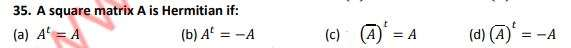46 / 58

The vertical lines of numbers in a matrix are called:

47 / 58

11. For the square matrix 𝑨 = [𝒂𝒊𝒋]𝒏×𝒏 then 𝒂𝟏𝟏, 𝒂𝟐𝟐, 𝒂𝟑𝟑…𝒂𝒏𝒏 are:

48 / 58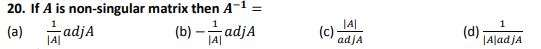49 / 58

If a matrix A has same number of rows and columns then A is called:

50 / 58

If a matrix A has only one column then it is called:

51 / 58

13. For a square matrix 𝑨 = [𝒂𝒊𝒋]if all 𝒂𝒊𝒋 = 𝟎, 𝒊 ≠ 𝒋 and at least 𝒂𝒊𝒋 ≠ 𝟎, 𝒊 = 𝒋 then A is called:

52 / 58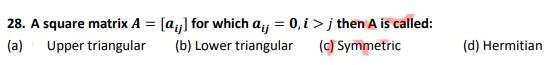53 / 58

For any matrix A , it is always true that

54 / 58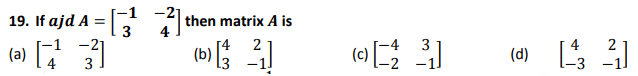55 / 58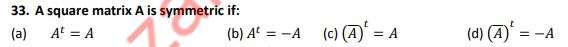56 / 58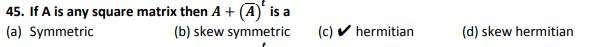57 / 58

5. The element 𝒂𝒊𝒋 of any matrix A is present in:

58 / 58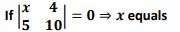The average score is 65%

0%

### One response to “FSC Mathematics MCQs Chapter 3”

1. Hamdan amin says:

This is very good MashAllah.. nice work

Salam